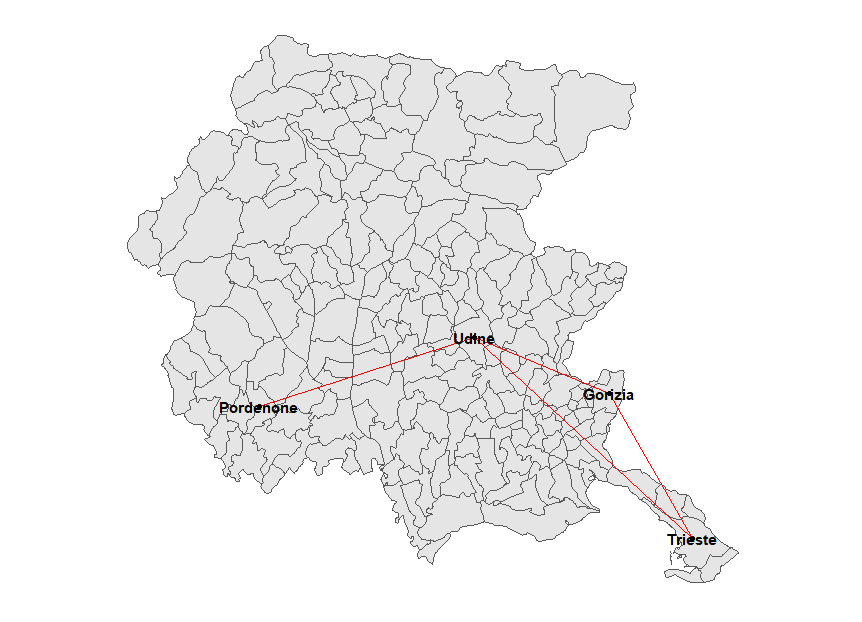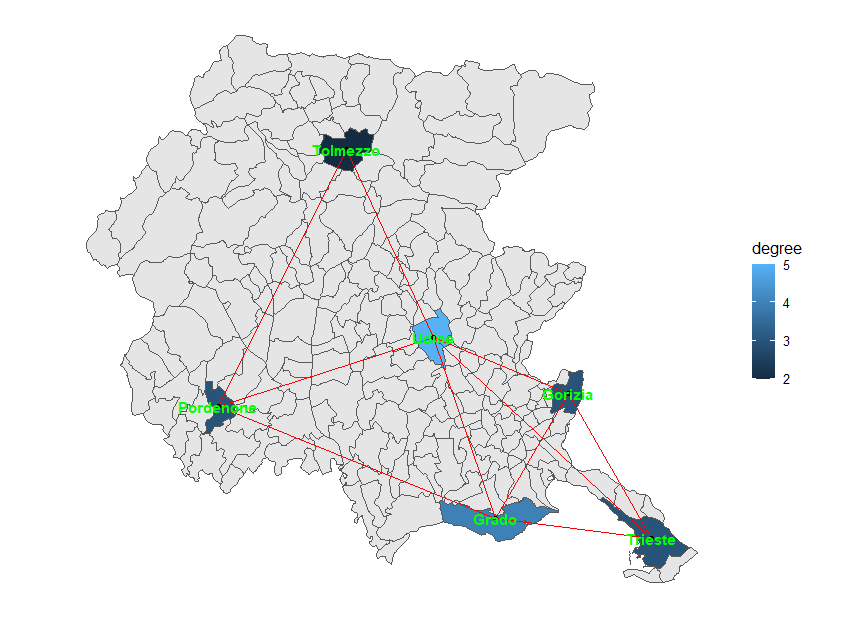# netmap: representing spatial networks with ggplot2

## Introduction

Some data sets are relational data sets, they represent relations between their units and which unit is connected to which ones, with a wide range of reasons for two units to be considered linked or not (family/kinship, trade, membership in a club etc.). Other data sets have a geographical, spatial component: each unit represents a part of the physical world, like a river, a city, a country, a landmark. Some data sets are both: relational data sets (networks) can be about units that have an inherent spatial aspect - trade networks between regions, relationships between users for which location data is available, traffic relationships between areas and so on. Network visualization is hard and most methods to visualize a network focus on displaying vertices and edges (loosely speaking, units of the network and relationships between them, respectively) in a way that highlights the properties of the network itself. When vertices already have a spatial component, though, a “natural” visualization is just to plot the vertices in their spatial position and display the edges between them. This may make the network more understandable, though not necessarily easier to read.

The netmap package doesn’t attempt to reinvent the wheel, so it uses both the sf package to handle spatial data files (from shapefiles to KML files to whatnot) and the ggnetwork package to plot network data using ggplot2’s grammar of graphics. It will work with network objects as produced by either the network or the igraph package, without the need to specify the object class. Elements of the sf objects are called features (i.e. a city, a state of a country), while network objects have vertices (the elements that may or may not be linked to each other) and edges (the connections between the vertices).

## Installation

The stable version of the package can be installed from CRAN:

install.packages("netmap")

devtools::install_github("artod83/netmap")

## Basic syntax

The main function is ggnetmap. It will need both a network object and a sf object and it will produce a data frame (a fortified data frame, as produced by fortify.network or fortify.igraph in ggnetwork). It will most commonly used like this (net is a network or igraph object, map a sf object, lkp_tbl is a lookup table, in case there is no direct match between the two objects, m_name and n_name are the variable names for linking the two objects):


fortified_df=ggnetmap(net, map, lkp_tbl, m_name="spatial_id", n_name="vertex.names")
ggplot() +
geom_sf(data=map) + #this will be the map on which the network will be overlayed
geom_edges(data=fortified_df, aes(x=x,y=y, xend=xend, yend=yend), colour="red") + #network edges
geom_nodes(data=fortified_df, aes(x=x,y=y)) + #network vertices
geom_nodetext(data=fortified_df, aes(x=x,y=y, label = spatial_id), fontface = "bold") + #vertex labels
theme_blank()

The data frame returned by ggnetmap will describe the edges of the network, from which vertex information can also be derived. It will contain both vertex identifiers (from the network and the sf object), the coordinates of the edge’s start (which will coincide with the vertex identifier) and the coordinates of the edge’s end. Since both identifiers are included, it is rather straightforward to add further variables by merging with other data frames.

The plot itself, it is based on two different data sources, the sf object and the data frame produced by ggnetmap , the latter overlayed to the former. We are trying to represent a network by using the spatial component of its vertices, so ggnetmap will only include elements that are both vertices of the network and features of the sf object, that is, the intersection between the set of the network vertices and the set of geographical features.

## Examples

An actual example would look like this:

library(ggplot2)
library(netmap)
data(fvgmap)
routes=network::network(matrix(c(0, 1, 1, 0,
1, 0, 1, 0,
1, 1, 0, 1,
0, 0, 1, 0), nrow=4, byrow=TRUE))
network::set.vertex.attribute(routes, "names", value=c("Trieste", "Gorizia", "Udine", "Pordenone"))
routes_df=netmap::ggnetmap(routes, fvgmap, m_name="Comune", n_name="names")
ggplot() +
geom_sf(data=fvgmap) +
ggnetwork::geom_edges(data=routes_df, aes(x=x,y=y, xend=xend, yend=yend), colour="red") +
ggnetwork::geom_nodes(data=routes_df, aes(x=x,y=y)) +
ggnetwork::geom_nodetext(data=routes_df, aes(x=x,y=y, label = Comune), fontface = "bold") +
theme_blank()Further aesthetics can be passed to geom_edges, geom_nodes and geom_nodetext, like different line types based on edge attributes.

When analyzing a network, measures of centrality like degree, betweenness and closeness are often used. The netmap package offers a convenient way of obtaining these centrality measures, with the ggcentrality function. This creates an sf object that acts as an additional layer that can be combined with the background sf object and the network visualization itself, as the following example shows:

routes2=network::network(matrix(c(0, 1, 1, 0, 0, 1 ,
1, 0, 1, 0, 0, 1,
1, 1, 0, 1, 1, 1,
0, 0, 1, 0, 1, 1,
0, 0, 1, 1, 0, 0,
1, 1, 1, 1, 0, 0), nrow=6, byrow=TRUE))
network::set.vertex.attribute(routes2, "names",
value=c("Trieste", "Gorizia", "Udine", "Pordenone",
lkpt=data.frame(Pro_com=c(32006, 31007, 30129, 93033, 30121, 31009),
names=c("Trieste", "Gorizia", "Udine", "Pordenone", "Tolmezzo",
routes2_df=netmap::ggnetmap(routes2, fvgmap, lkpt, m_name="Pro_com", n_name="names")
map_centrality=netmap::ggcentrality(routes2, fvgmap, lkpt, m_name="Pro_com",
n_name="names", par.deg=list(gmode="graph"))
ggplot() +
geom_sf(data=fvgmap) +
geom_sf(data=map_centrality, aes(fill=degree)) +
ggnetwork::geom_edges(data=routes2_df, aes(x=x,y=y, xend=xend, yend=yend), colour="red") +
ggnetwork::geom_nodes(data=routes2_df, aes(x=x,y=y)) +
ggnetwork::geom_nodetext(data=routes2_df, aes(x=x,y=y, label = names), fontface = "bold") +
theme_blank()While the above example shows the degree of the vertices, different centrality measures can be represented just by changing the aesthetic.

## Basic plotting

It’s also possible to plot just the network using the geographical position of the nodes as layout without using ggplot2, but instead resorting to the plot.network and plot.igraph functions. In this case, the layout function network.layout.extract_coordinates should be passed to the plotting functions or the wrapper netmap_plot should be used instead, as in the following example. Note that the features and the vertices should have the same order for network.layout.extract_coordinates to produce correct results; otherwise, use netmap_plot. Please also note that it’s not possible to overlay the network on the map this way.

routes2=network::network(matrix(c(0, 1, 1, 0, 0, 1 ,
1, 0, 1, 0, 0, 1,
1, 1, 0, 1, 1, 1,
0, 0, 1, 0, 1, 1,
0, 0, 1, 1, 0, 0,
1, 1, 1, 1, 0, 0), nrow=6, byrow=TRUE))
network::set.vertex.attribute(routes2, "names",
value=c("Trieste", "Gorizia", "Udine", "Pordenone",
netmap::netmap_plot(routes2, fvgmap, lkpt, m_name="Pro_com", n_name="names")